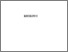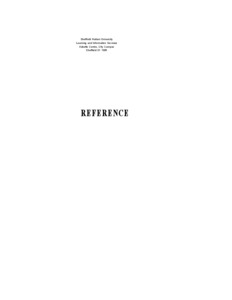# 3D modelling using partial differential equations (PDEs).

OSMAN, Abdusslam. (2014). 3D modelling using partial differential equations (PDEs). Doctoral, Sheffield Hallam University (United Kingdom)..Preview
PDF (Version of Record)
10697460.pdf - Accepted Version

Related URLs:

## Abstract

Partial differential equations (PDEs) are used in a wide variety of contexts in computer science ranging from object geometric modelling to simulation of natural phenomena such as solar flares, and generation of realistic dynamic behaviour in virtual environments including variables such as motion, velocity and acceleration. A major challenge that has occupied many players in geometric modelling and computer graphics is the accurate representation of human facial geometry in 3D. The acquisition, representation and reconstruction of such geometries are crucial for an extensive range of uses, such as in 3D face recognition, virtual realism presentations, facial appearance simulations and computer-based plastic surgery applications among others. The principle aim of this thesis should be to tackle methods for the representation and reconstruction of 3D geometry of human faces depending on the use of partial differential equations and to enable the compression of such 3D data for faster transmission over the Internet. The actual suggested techniques are based on sampling surface points at the intersection of horizontal and vertical mesh cutting planes. The set of sampled points contains the explicit structure of the cutting planes with three important consequences: 1) points in the plane can be defined as a one dimensional signal and are thus, subject to a number of compression techniques; 2) any two mesh cutting planes can be used as PDE boundary conditions in a rectangular domain; and 3) no connectivity information needs to be coded as the explicit structure of the vertices in 3D renders surface triangulation a straightforward task. This dissertation proposes and demonstrates novel algorithms for compression and uncompression of 3D meshes using a variety of techniques namely polynomial interpolation, Discrete Cosine Transform, Discrete Fourier Transform, and Discrete Wavelet Transform in connection with partial differential equations. In particular, the effectiveness of the partial differential equations based method for 3D surface reconstruction is shown to reduce the mesh over 98.2% making it an appropriate technique to represent complex geometries for transmission over the network.

Item Type: Thesis (Doctoral) Thesis (Ph.D.)--Sheffield Hallam University (United Kingdom), 2014. Sheffield Hallam Doctoral Theses EPrints Services 10 Apr 2018 17:21 26 Apr 2021 11:19 https://shura.shu.ac.uk/id/eprint/20153View Item